# Math in Focus Grade 1 Chapter 1 Answer Key Numbers to 10

This handy Math in Focus Grade 1 Workbook Answer Key Chapter 1 Numbers to 10 detailed solutions for the textbook questions.

## Math in Focus Grade 1 Chapter 1 Answer Key Numbers to 10

challenging Practice

Mother Hen’s eggs have numbers that are greater than 2 and less than 8.

Color the eggs that belong to Mother Hen.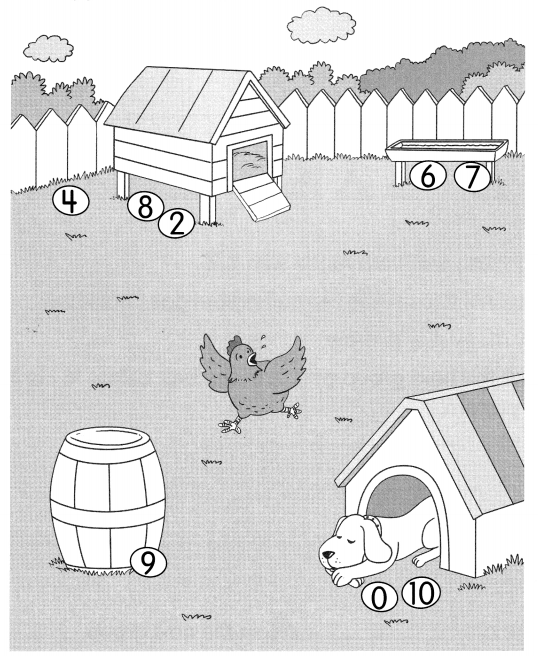Explanation: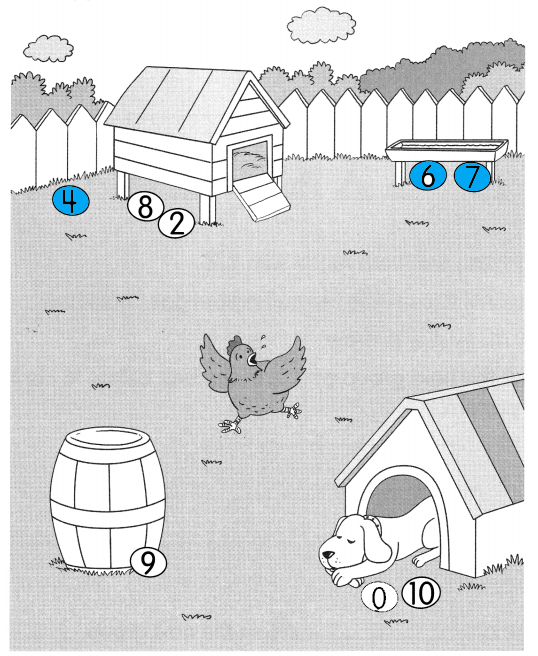The numbers greater than 2 and lesser than 8 are 3, 4, 5, 6, 7,
The eggs with 4 , 6 and 7 are shaded

Problem Solving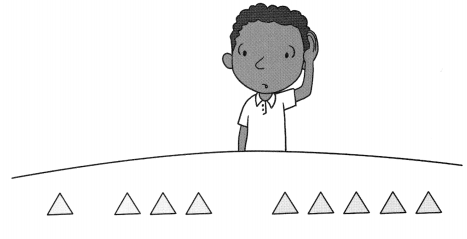Daryl sees a pattern made with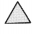.
He wants to continue the pattern but does not know how manyto draw.
Draw the next group ofin the box below to continue the pattern.
______________
There are ___in the next group.
In The next pattern there will be 7 triangles
1 after 3
3 after 5 and 5 after 7There are 7in the next group.

Chapter Review/Test
Vocabulary

Match.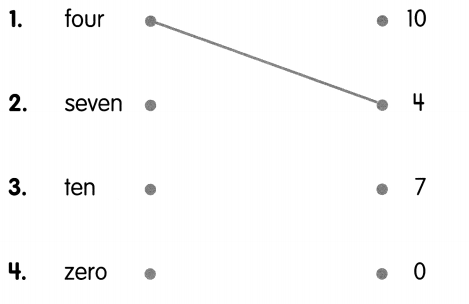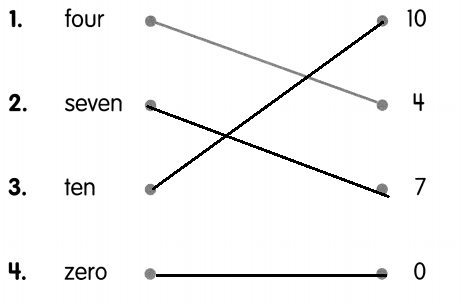Explanation:
The given number names are matched with the given numbers

Concepts and Skills

Circle the stars to show the number. Write the number in words.

Question 5.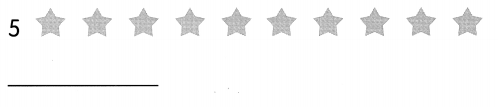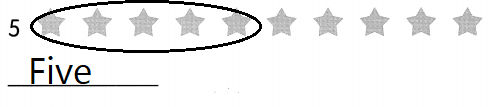Explanation:
The given number is 5
so, circled the five stars and the number name of 5 is five

Question 6.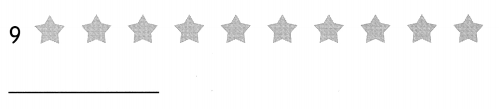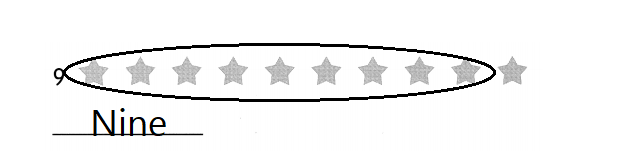Explanation:
the given number is 9
so, circled the nine stars
The number name of 9 is nine.

Fill in the blanks with greater than, less than or the same as.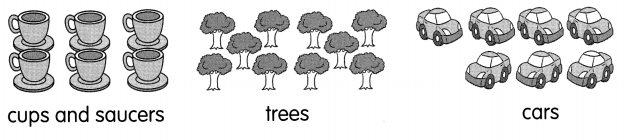Question 7.
The number of cups is ____ the number of saucers.
Same
Explanation:
There are 6 cups and 6 saucers
6 = 6

Question 8.
The number of trees is ____ the number of cars.
Greater than
Explanation:
There are 10 trees and the number of  cars are 7
10 > 7

Question 9.
The number of cars is ___ the number of cups.
Greater than
Explanation:
Number of cars are 7 and number of cups are 6
7 > 6

Write any two numbers.

Question 10.
greater than 5: ____ _____.
6 and 7
Explanation:
5 + 1 = 6
6 + 1 = 7
The numbers greater than 5 are 6 and 7

Question 11.
less than 7: ____ _____
6 and 5
Explanation:
7 – 1 = 6
6 – 1 = 5
The number lesser than 7 is 6 and 5

Write the missing numbers in the number pattern.

Question 12.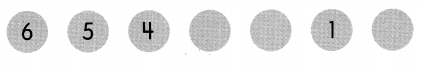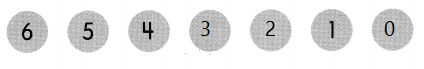Explanation:
The missing numbers are 3, 2, 1
And the given pattern is in greater number to lesser numbers.

Question 13.
4 is 1 less than ____